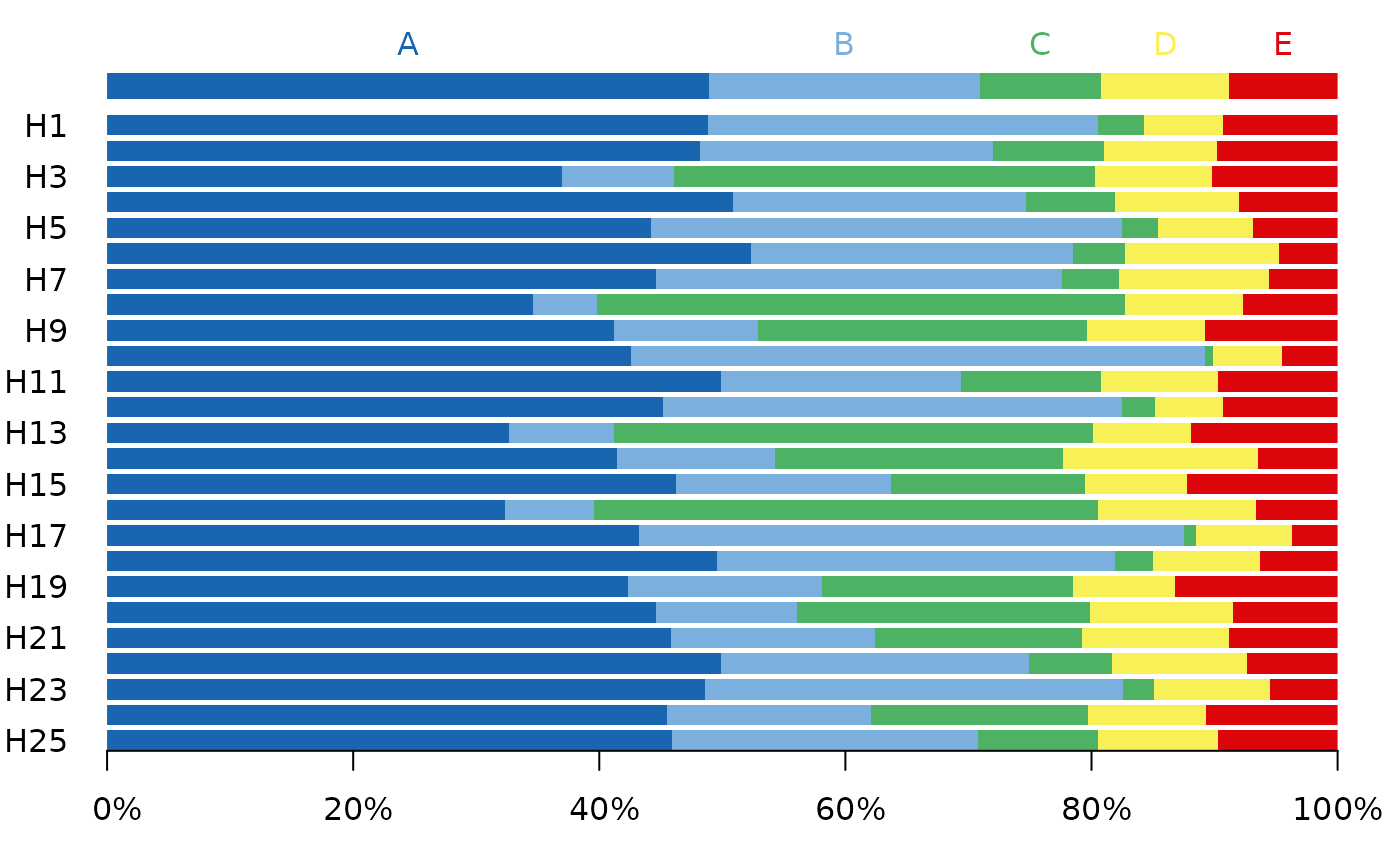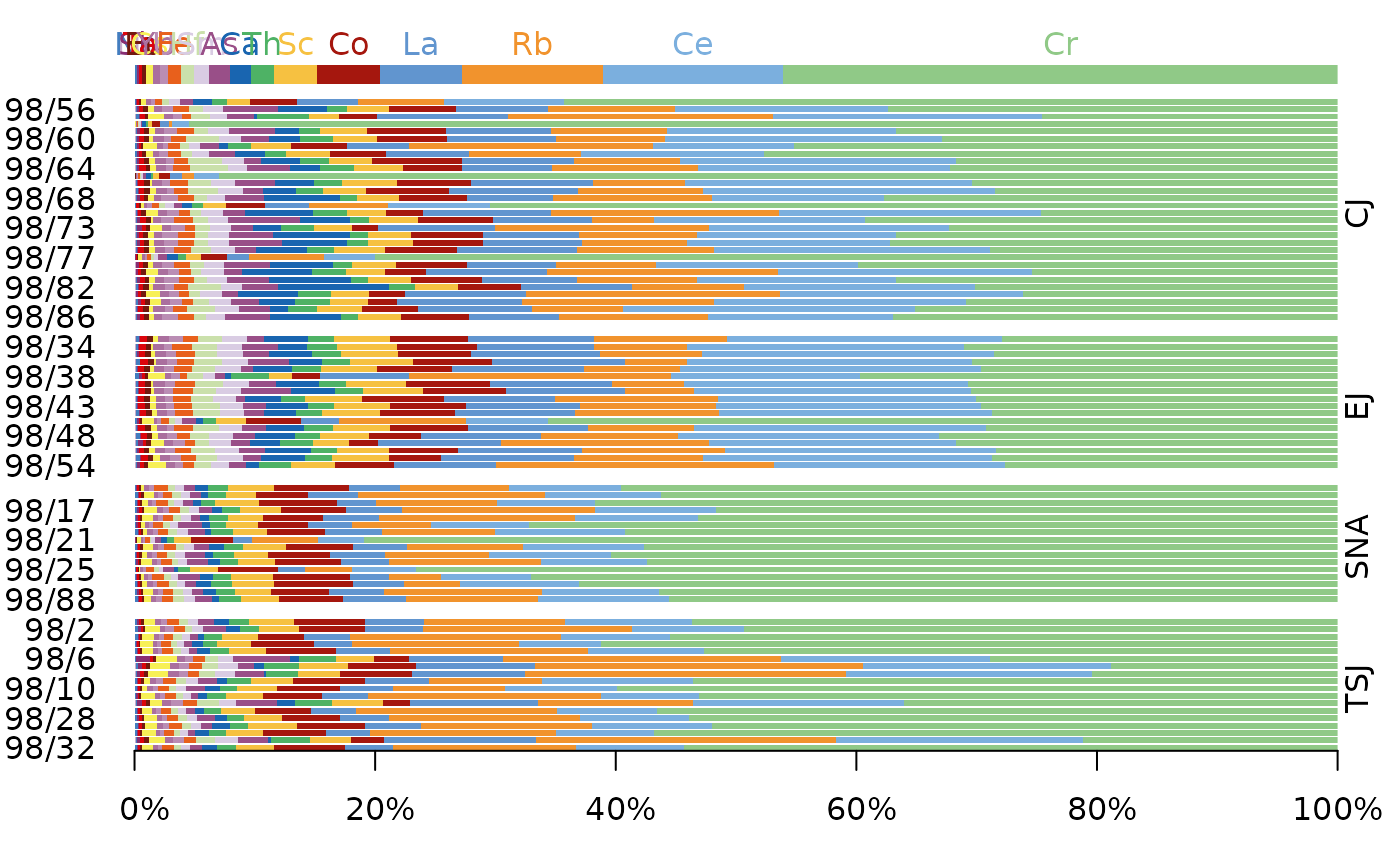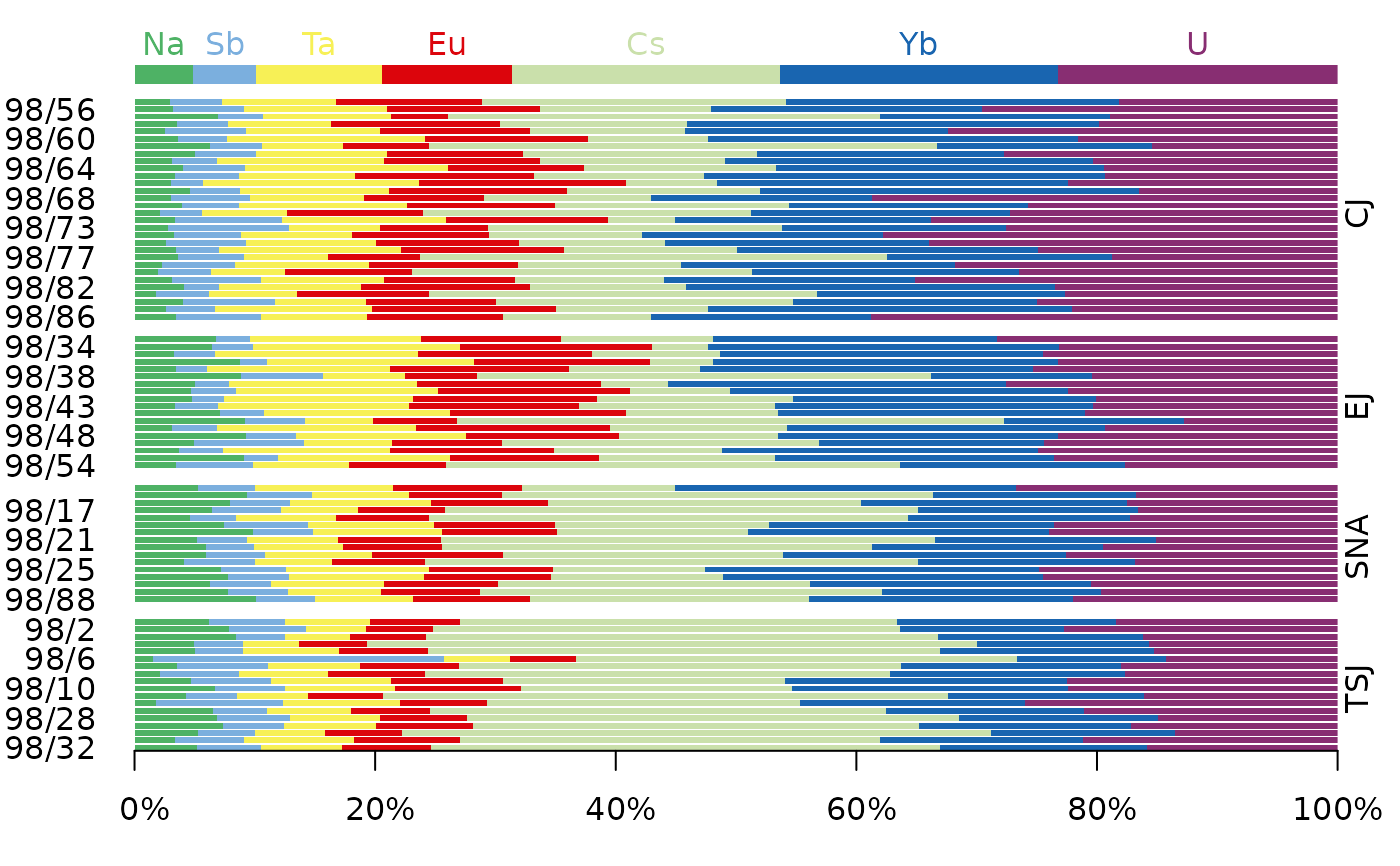Displays a compositional bar chart.

## Usage

# S4 method for CompositionMatrix
barplot(
height,
order = NULL,
decreasing = FALSE,
groups = get_groups(height),
horiz = TRUE,
xlab = NULL,
ylab = NULL,
main = NULL,
sub = NULL,
ann = graphics::par("ann"),
axes = TRUE,
...
)

## Arguments

height

A CompositionMatrix object.

order

An integer vector giving the index of the column to be used for the ordering of the data.

decreasing

A logical scalar: should the sort order be increasing or decreasing?

groups

A factor in the sense that as.factor(groups) defines the grouping. If set, a matrix of panels defined by groups will be drawn.

horiz

A logical scalar. If FALSE, the bars are drawn vertically with the first bar to the left. If TRUE (the default), the bars are drawn horizontally with the first at the bottom.

xlab, ylab

A character vector giving the x and y axis labels.

main

A character string giving a main title for the plot.

sub

A character string giving a subtitle for the plot.

ann

A logical scalar: should the default annotation (title and x and y axis labels) appear on the plot?

axes

A logical scalar: should axes be drawn on the plot?

...

Further parameters to be passed to graphics::barplot().

## Value

barplot() is called for its side-effects: is results in a graphic being displayed (invisibly return height).

Other plot methods: as_graph(), plot_logratio

N. Frerebeau

## Examples

## Coerce to compositional data
data("hongite")
coda <- as_composition(hongite)

## Bar plot
barplot(coda, order = 2)## Data from Day et al. 2011
data("kommos", package = "folio") # Coerce to compositional data
kommos <- remove_NA(kommos, margin = 1) # Remove cases with missing values
coda <- as_composition(kommos, groups = 1) # Use ceramic types for grouping
#> 1 qualitative variable was removed: date.

barplot(coda, order = 1, main = "Kommos Ceramics")barplot(coda, order = 1, horiz = FALSE, main = "Kommos Ceramics")